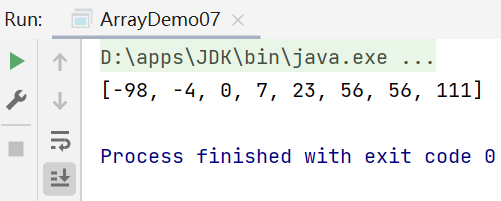# day08-Java数组

2022-07-27 19:32:31  阅读：29  来源： 互联网

# Java数组

## 1.数组概述

• 数组是相同类型数据的有序集合
• 数组描述的是相同类型的若干个数据，按照一定的先后次序排列组合而成
• 其中每一个数据称作一个数组元素，每个数组元素可以通过一个下标来访问它们

## 2.数组的声明和创建

• 首先必须声明数组变量，才能在程序中使用数组。下面是声明数组变量的语法：、

``````dataType[] arrayRefVar;	//首选的方法
或
dataType arrayRefVar[];	//效果相同，但不是首选方法
``````
• Java语言使用new操作符来创建数组，语法如下：

``````dataType[] arrayRefVar = new dataType[arraySize];
``````
• 数组的元素是通过索引访问的，数组索引从0开始

• 获取数组长度：arrays.length

``````package li.bolog.array;

public class ArrayDemo01 {
//变量的类型 变量的名字   =   变量的值
//数组类型
public static void main(String[] args) {
int[] nums;//1.声明一个数组

nums = new int;//2.创建一个数组
//或者整合起来：int[] nums = new int;

//3.给数组元素赋值,数组元素下标从0开始
nums=1;
nums=2;
nums=3;
nums=4;
nums=5;
nums=6;
nums=7;
nums=8;
nums=9;
nums=10;
System.out.println(nums);//10

//计算所有元素的和
int sum=0;
//获取数组长度：arrays.length
for (int i = 0; i < nums.length; i++) {
sum=sum+nums[i];
}
System.out.println("总和为："+sum);
}
}
``````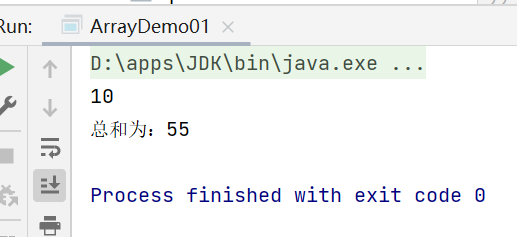## 3.三种初始化及内存分析

### 3.1内存分析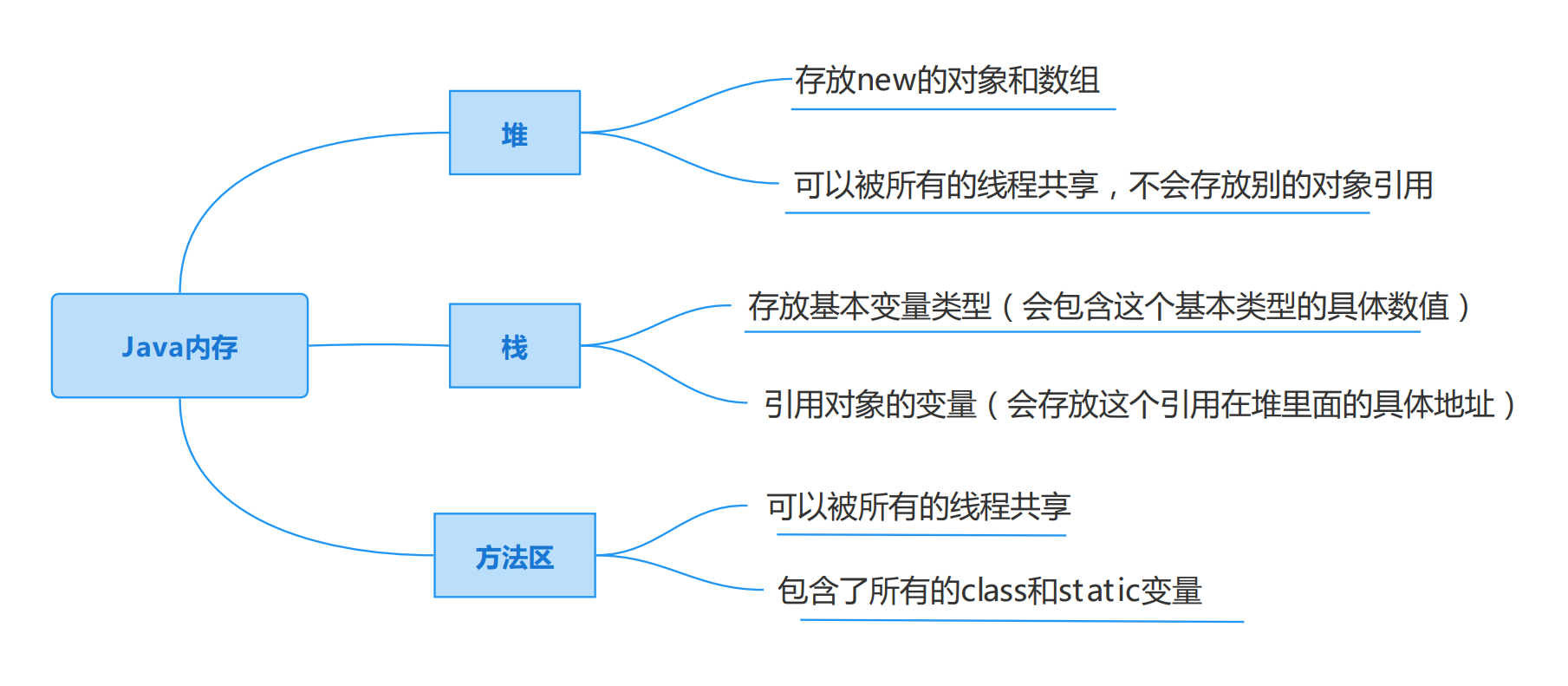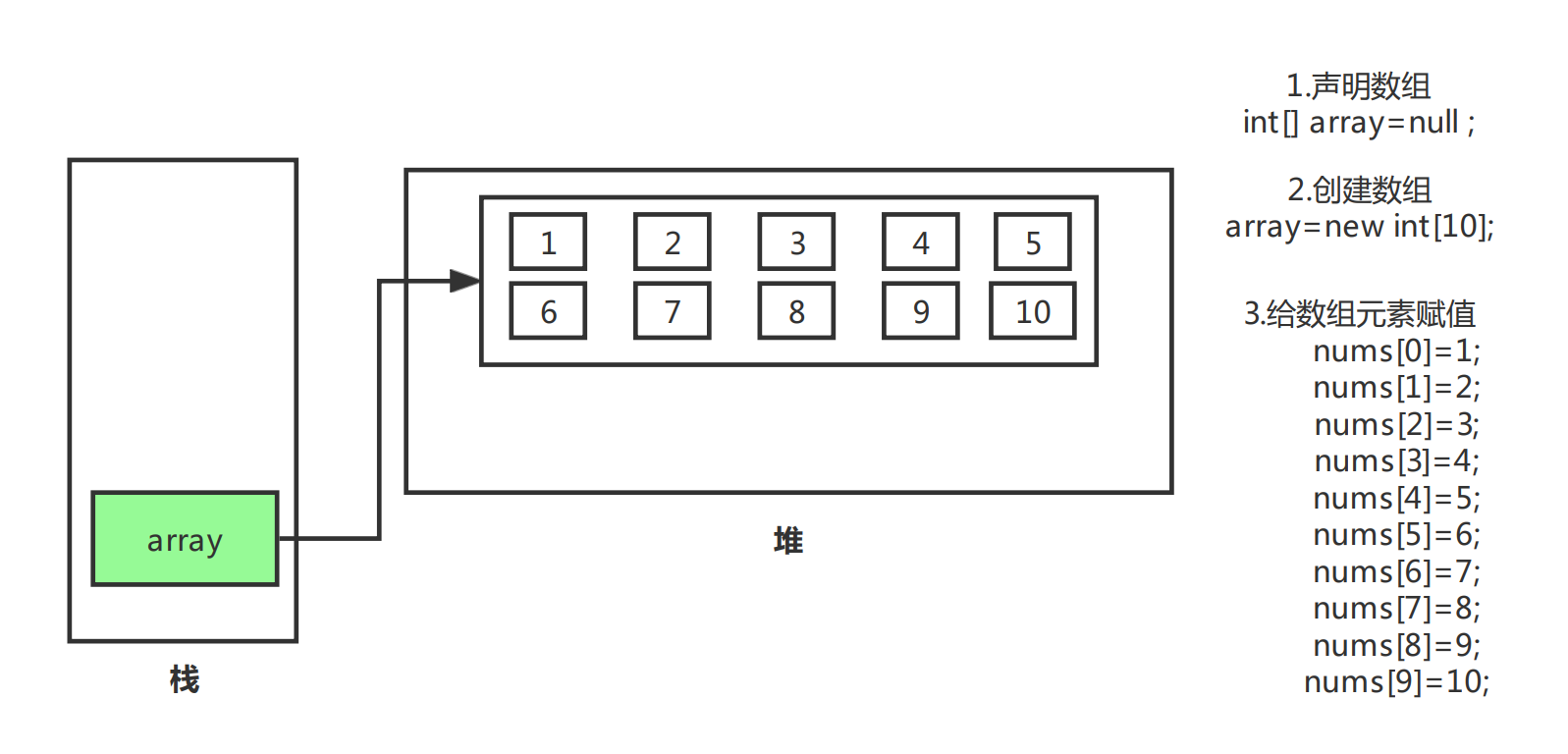### 3.2三种初始化

• 静态初始化
``````int[] a= {1,2,3};
Man[] mans = {new Man(1,1),new Man(2,2)};
``````
• 动态初始化
``````int[] a=new int;
a=1;
a=2;
``````
• 数组的默认初始化
• 数组是引用类型，它的元素相当于类的实例变量，因此数组已经一经分配空间，其中的每个元素也被按照实例变量同样的方式被隐式初始化

``````package li.bolog.array;

public class ArrayDemo02 {
public static void main(String[] args) {
//静态初始化：创建+赋值
int[] a={1,2,3,5,89};

//动态初始化:包含默认初始化，int类型默认值为0，string类型默认值为null
int[] b= new int;
b=10;
System.out.println(b);//10
System.out.println(b);//输出默认值0
System.out.println(b);//输出默认值0
System.out.println(b);//输出默认值0

}
}
``````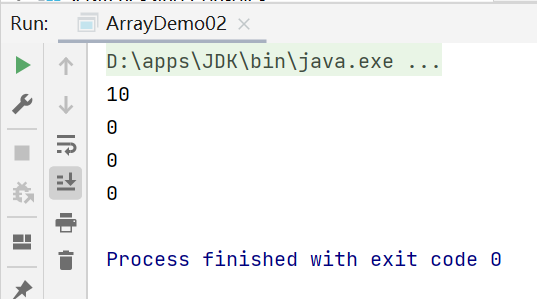## 4.下标越界及小结

### 4.1数组的四个基本特点

• 其长度是确定的。数组一旦被创建，它的大小就是不可以被改变的
• 其元素必须是相同类型，不允许出现混合类型
• 数组中的元素可以是任何数据类型，包括基本数据类型和引用数据类型
• 数组变量属于引用类型，数组也可以看成是对象，数组中的每个元素相当于该对象的成员变量。数组本身就是对象，Java对象是在对中的，因此数组无论保存原始类型还是其他对象类型，数组本身是在堆中的

### 4.2数组边界

• 下标的合法区间：[0,length-1]，如果越界就会报错
``````public static void main(String[] args){
int[] a=new int;
System.out.println(a);  //越界
}
``````
``````报错：ArrayIndexOutOfBoundsException：数组下标越界异常
``````
• 小结：
• 数组是相同数据类型（数据类型可以是任意类型）的有序集合
• 数组也是对象。数组元素相当于对象的成员变量
• 数组的长度是确定的，不可变的。如果越界则会报错：ArrayIndexOutOfBoundsException

## 5.数组的使用

• for-each循环
• 数组作方法入参
• 数组作返回值

``````package li.bolog.array;

public class ArrayDemo03 {
public static void main(String[] args) {
int[] arrays = {1,2,3,4,5} ;

//打印所有的数组元素
for (int i = 0; i < arrays.length; i++) {
System.out.println(arrays[i]);
}
System.out.println("===========");

//计算数组所有元素的和
int sum=0;
for (int i = 0; i < arrays.length ; i++) {
sum = sum + arrays[i] ;
}
System.out.println("sum:"+sum);
System.out.println("===========");

//查找最大元素
int max = arrays; ;
for (int i = 1; i < arrays.length; i++) {
if (max < arrays[i]) {
max=arrays[i];
}

}
System.out.println("max:"+max);
}
}
``````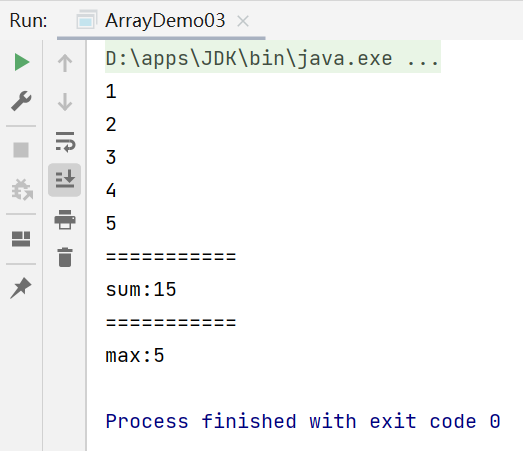``````package li.bolog.array;

public class ArrayDemo04 {
public static void main(String[] args) {
int[] arrays={1,2,3,4,5};

for (int array : arrays) {
System.out.println(array);
}
}
}
``````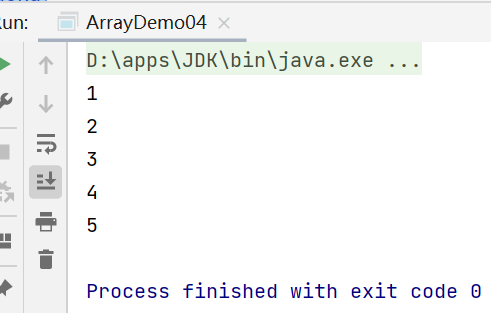``````package li.bolog.array;

public class ArrayDemo04 {
public static void main(String[] args) {
int[] arrays={1,2,3,4,5};

printArray(arrays);

System.out.println();

int[] reverse = reverse(arrays);
printArray(reverse);

}

//打印数组元素
public static void printArray(int[] arrays){
for (int i = 0; i < arrays.length; i++) {
System.out.print(arrays[i]+"\t");
}
}

//数组作为返回值
//反转数组
public static int[] reverse(int[] arrays){
int[] result = new int[arrays.length];

for (int i = 0,j= result.length-1; i < arrays.length; i++,j--) {
result[j]=arrays[i];
}
return result;
}
}
``````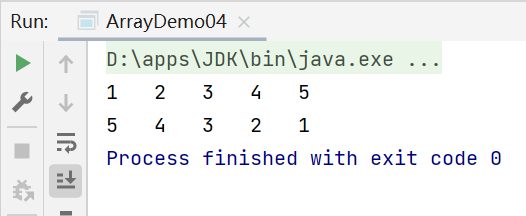## 6.多维数组

• 多维数组可以看成是数组的数组，如一个二维数组就是一个特殊的一维数组，每一个元素都是一个一维数组
• 二维数组
``````int a[][]=new int;
``````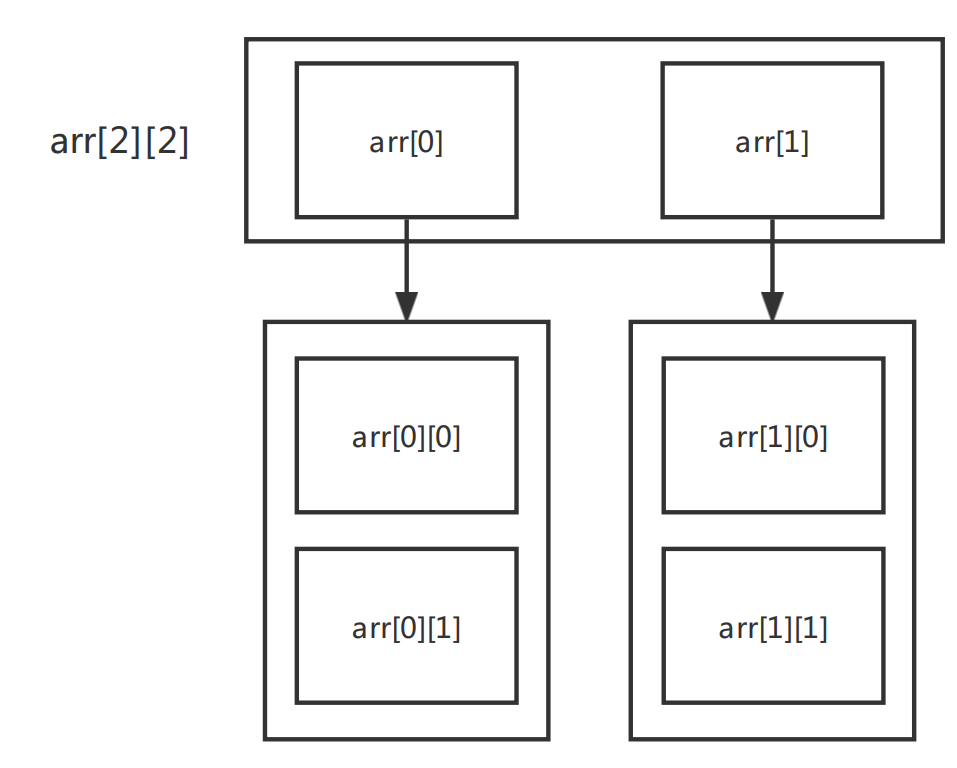``````package li.bolog.array;

public class ArrayDemo05 {
public static void main(String[] args) {

int[][] array={{1,2},{2,3},{3,4},{4,5}};//
/*
1,2     array
2,3     array
3,4     array
4,5     array
*/

System.out.println(array);
System.out.println(array);

//二维数组的长度
System.out.println(array.length);//4,表示外层
System.out.println(array.length);//2,表示里层

for (int i = 0; i < array.length; i++) {//外层
for (int j = 0; j < array[i].length; j++) {//里层
System.out.print(array[i][j]+"\t");

}

}

}
}
``````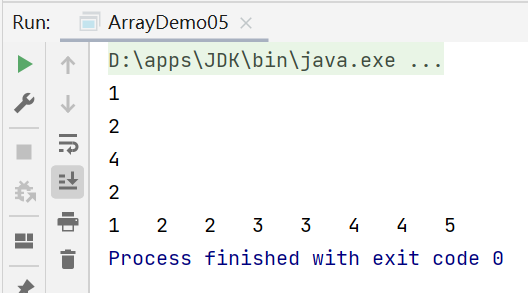## 7.Arrays类

• 数组的工具类java.util.Arrays
• 由于数组对象本身并没有什么方法可以供我们使用，但API中提供了一个工具类Arrays供我们使用，从而可以对数据对象进行一些基本的操作
• 查看JDK帮助文档
• Array类中的方法都是stasic修饰的静态方法，在使用的时候可以直接使用类名进行调用，而“不用”使用对象进行调用（注意：是“不用”而不是“不能”）
• 具有以下常用功能：
• 给数组赋值：通过fill方法
• 对数组排序：通过sort方法，按升序
• 比较数组：通过equals方法比较数组中元素值是否相等
• 查找数组元素：通过binarySearch方法能对排序号的数组进行二分查找法操作

``````package li.bolog.array;

import java.util.Arrays;

public class ArrayDemo06 {
public static void main(String[] args) {
int[] a={1,2,3,4,9090,543,21,3,23};

System.out.println(a);//[I@1b6d3586

System.out.println(Arrays.toString(a));
printArray(a);
}

//重复造轮子（打印数组元素）：Arrays.toString()
public static void printArray(int[] a){
for (int i = 0; i < a.length; i++) {
if(i==0){
System.out.print("[");
}
if (i==a.length-1){
System.out.print(a[i]+"]");
}else {
System.out.print(a[i]+", ");
}
}

}
}
``````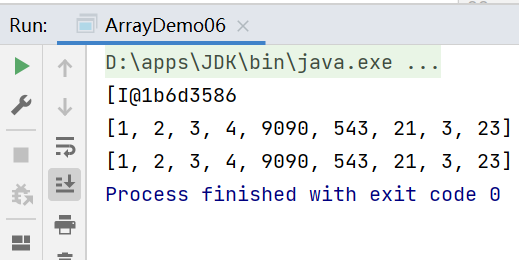``````package li.bolog.array;

import java.util.Arrays;

public class ArrayDemo06 {
public static void main(String[] args) {
int[] a={1,2,3,4,9090,543,21,3,23};

Arrays.sort(a);
System.out.println(Arrays.toString(a));

}

}
``````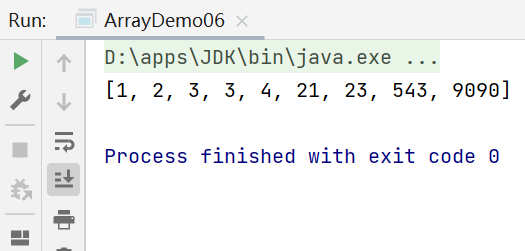``````package li.bolog.array;

import java.util.Arrays;

public class ArrayDemo06 {
public static void main(String[] args) {
int[] a={1,2,3,4,9090,543,21,3,23};

Arrays.sort(a);
System.out.println(Arrays.toString(a));

Arrays.fill(a,2,4,0);//将数组a中第二个到第四个数的值全部填充为0
System.out.println(Arrays.toString(a));

Arrays.fill(a,0);//将数组a中的值全部填充为0
System.out.println(Arrays.toString(a));

}

}
``````

## 8.冒泡排序

• 冒泡排序的算法：两层循环，外层冒泡轮数，里层依次比较。

• 时间复杂度为:
\$\$
O(n^2)
\$\$

``````package li.bolog.array;

//冒泡排序
//1.比较数组中两个相邻的元素，如果第一个数比第二个数大，我们就交换他们的位置
//2.每一次比较都会产生出一个最大或者最小的数字
//3.下一轮则可以少一次排序

import java.util.Arrays;

public class ArrayDemo07 {
public static void main(String[] args) {
int[] a={-4,56,7,56,23,111,0,-98};

int[] sort = sort(a);
System.out.println(Arrays.toString(sort));
}

public static int[] sort(int[] array) {
//临时变量
int temp=0;
//外层循环，判断要走多少次
for (int i = 0; i <array.length-1 ; i++) {
//内层循环，比较判断两个数，如果第一个数比第二个数大则交换位置

for (int j = 0; j < array.length-1-i; j++) {//a.length-1-i  是因为每一次比较都会产生出一个最大或者最小的数字，因此每一轮比较的次数都会少1
if(array[j+1]<array[j]){
temp=array[j];
array[j]=array[j+1];
array[j+1]=temp;
}
}
}
return array;
}
}
``````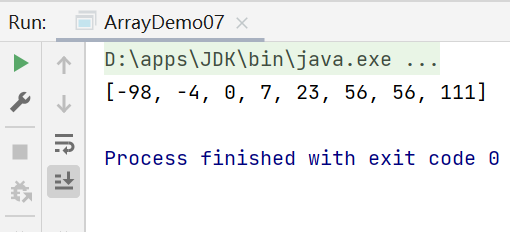``````package li.bolog.array;

//冒泡排序
//1.比较数组中两个相邻的元素，如果第一个数比第二个数大，我们就交换他们的位置
//2.每一次比较都会产生出一个最大或者最小的数字
//3.下一轮则可以少一次排序

import java.util.Arrays;

public class ArrayDemo07 {
public static void main(String[] args) {
int[] a={-4,56,7,56,23,111,0,-98};

int[] sort = sort(a);
System.out.println(Arrays.toString(sort));
}

public static int[] sort(int[] array) {
//临时变量
int temp=0;
//外层循环，判断要走多少次
for (int i = 0; i <array.length-1 ; i++) {
//内层循环，比较判断两个数，如果第一个数比第二个数大则交换位置

boolean flag=false;//通过flag标识位减少没有意义的比较，用于已经排好序的顺序

for (int j = 0; j < array.length-1-i; j++) {//a.length-1-i  是因为每一次比较都会产生出一个最大或者最小的数字，因此每一轮比较的次数都会少1
if(array[j+1]<array[j]){
temp=array[j];
array[j]=array[j+1];
array[j+1]=temp;
flag=true;
}
}
if(flag==false){//如果数组已经排好顺序，则可以结束运行
break;
}
}
return array;
}
}
``````